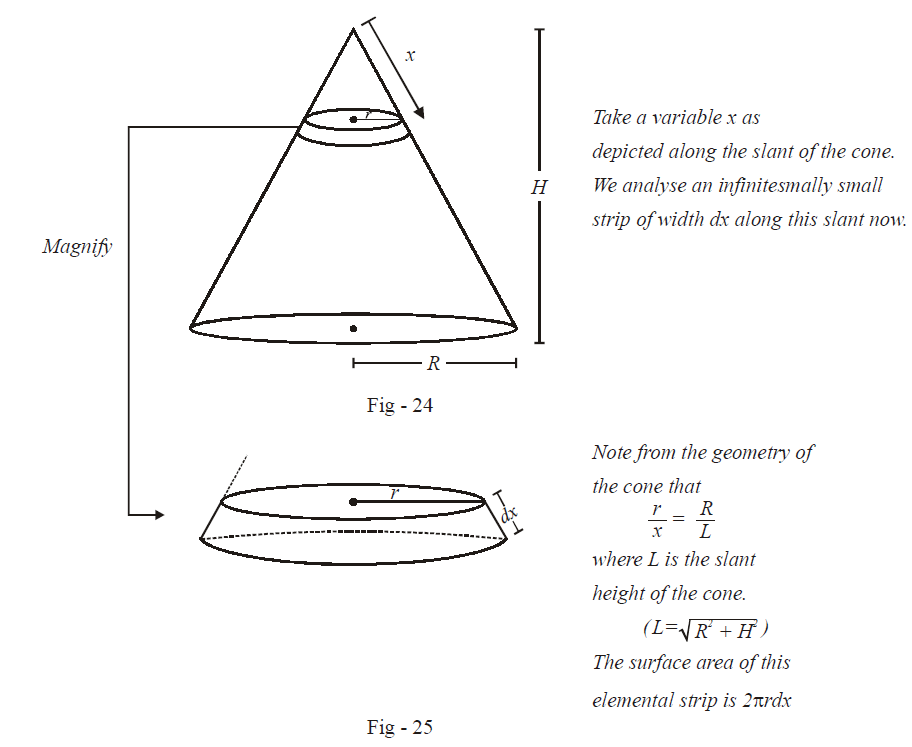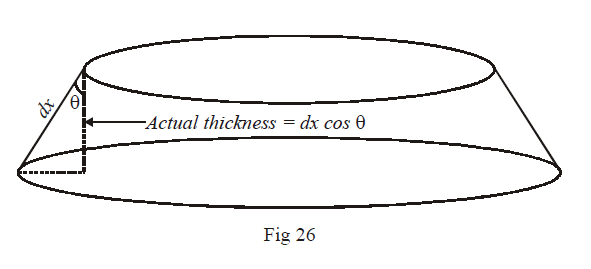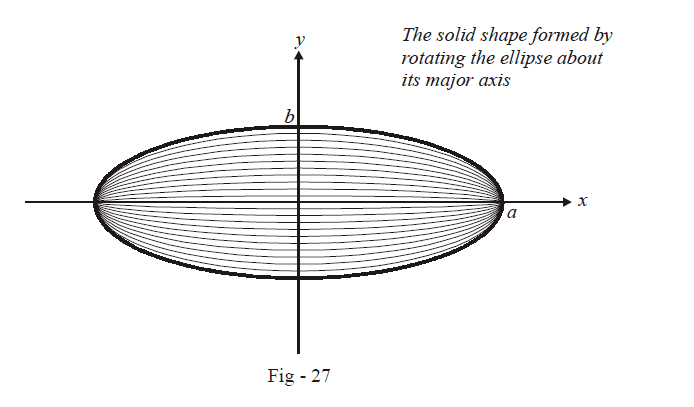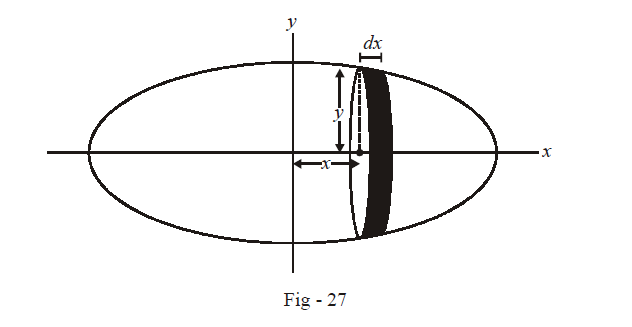# Problems On Volumes Set-1

Go back to  'Areas and Volumes'

Example – 19

Find the lateral surface area and the volume of a cone of height H and base radius R.

Solution: This question is an easy application question of definite integration. Let us evaluate the surface area first.From the explanation given in the figure:

\begin{align}{\rm{Lateral\;Surface\;Area}}\;A &= \int\limits_0^L {2\pi rd} \\ & = \frac{{2\pi R}}{L}\int\limits_0^L {xdx} \\ & = \pi RL\end{align}

To calculate the volume, we again use the elemental strip analysed in Fig -25. The thickness of this strip is not dx but dx where $$cos\theta = \frac{H}{L}$$The volume of this strip is therefore $$\pi {r^2}dx\cos \theta$$ .Thus, the total volume is

\begin{align}V& = \int\limits_0^L {\pi {r^2}dx\cos \theta }\\& = \frac{\pi }{L}.\frac{{{R^2}}}{{{L^2}}}\int\limits_0^L {{x^2}} dx\\&= \frac{{\pi {R^2}H}}{{{L^3}}}.\frac{{{L^3}}}{3}\\&= \frac{1}{3}\pi {R^2}H\end{align}

Example – 20

An ellipse \begin{align}\frac{{{x^2}}}{{{y^2}}} + \frac{{{y^2}}}{{{b^2}}} = 1\end{align} (where $$a > b$$)is rotated about its major axis. Find the volume generated

Solution: An ellipse, when rotated about its major axis, will form an “egg-shaped” volume. (It won’t be exactly egg-shaped though! It will be symmetric about its centre plane (a plane through the line $$y = 0$$ perpendicular to the $$x - y$$ plane) while an egg is not exactly symmetric in this way).We take an elemental strip of thickness $$dx$$ at a distance $$x$$ from the origin.As is clear from the figure, the volume of this elemental strip is

\begin{align}V&= \pi {y^2}dx\\&= \pi b\left( {1 - \frac{{{x^2}}}{{{a^2}}}} \right)dx\end{align}

Thus, the volume generated of the right half would be :

\begin{align}{V_{half}}&= \int\limits_0^a {dV = \int\limits_o^a {\pi {b^2}} \left( {1 - \frac{{{x^2}}}{{{a^2}}}} \right)} dx\\& = \pi {b^2}\left. {\left( {x - \frac{{{x^3}}}{{3{a^2}}}} \right)} \right|_0^a\\& = \pi {b^2}\left( {a - \frac{a}{3}} \right)\\ &= \frac{{2\pi a{b^2}}}{3}\end{align}

The total volume is therefore

$V = 2 \times {V_{half}} = \frac{{4\pi a{b^2}}}{3}$

Now, try calculating the surface area of this volume generated.

## TRY YOURSELF – 1

Q. 1   Show that the area of the ellipse $$\frac{{{x^2}}}{{{a^2}}} + \frac{{{y^2}}}{{{b^2}}} = 1\;{\rm{ is}}{\rm{ }}\;\pi ab$$

Q. 2   If $${S_1} = \int\limits_0^{2\pi } {\max \left( {\sin x,\cos x} \right)}\;dx\;and\;{S_1} = \int\limits_0^{2\pi } {\min \left( {\sin x,\cos x} \right)} dx$$ , show that $${S_1} = {S_2}$$ .

Q. 3   Prove that the area of the region represented by $\left| {x - 2y} \right| + \left| {x + 2y} \right| \le 8\;{\rm{ and}}\;xy \ge 2{\rm{ }}\;is{\rm{ }}\;4(3 - ln{\rm{ }}4).$

Q. 4   Prove that the area enclosed by the region $$R \equiv \left\{ {\left( {x,y} \right):{y^2} \le x \le \left| y \right|} \right\}\;\rm{is}\;\frac{1}{3}$$ .

Q. 5   Find the surface area of the volume generated by rotating the ellipse $$\frac{{{x^2}}}{{{a^2}}} + \frac{{{y^2}}}{{{b^2}}} = 1{\rm{(a > b)}}$$ about its major axis. Hence find the surface area of a sphere of radius r.